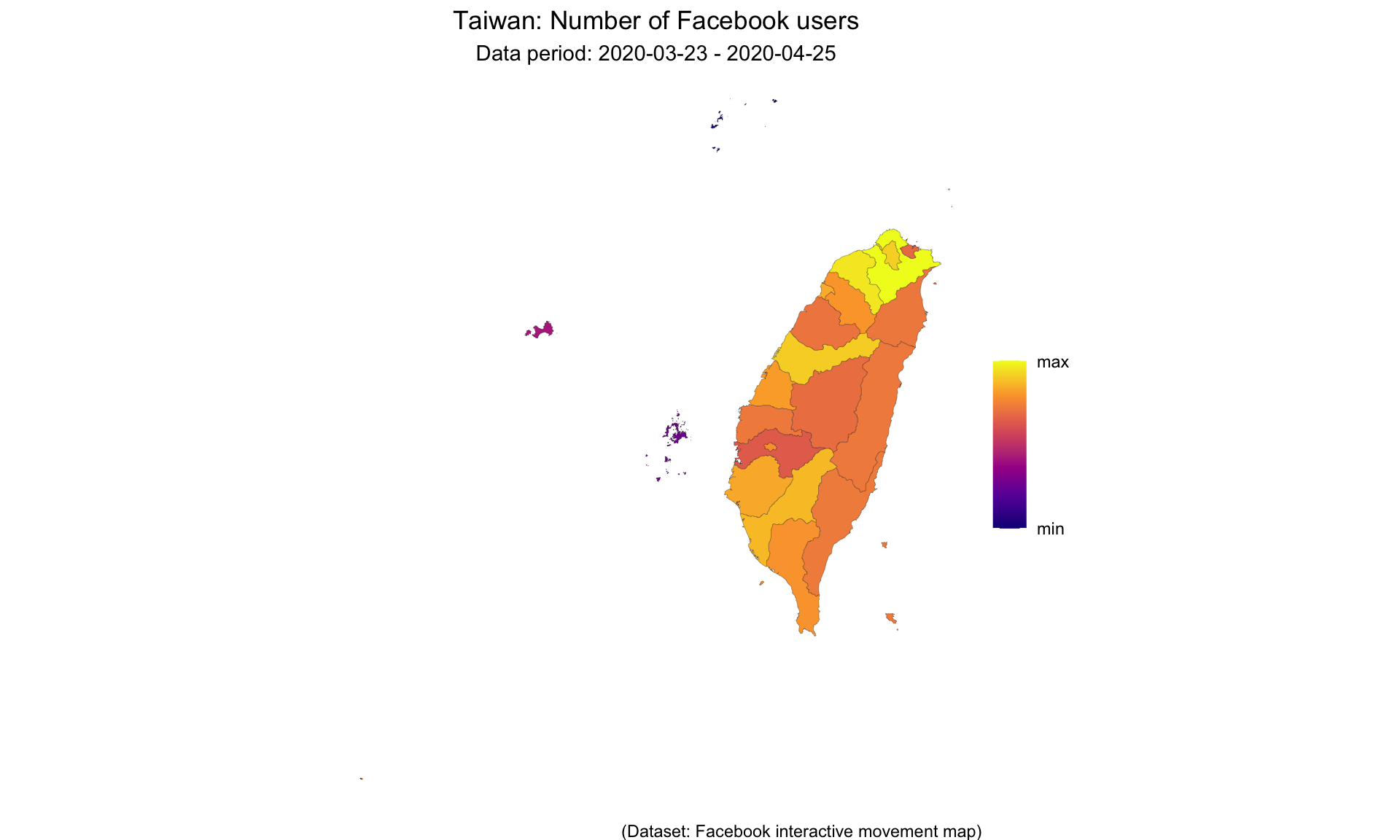## Taiwan

``````#knitr::opts_chunk\$set(cache.path = "../cache_reports/")
#knitr::opts_chunk\$set(cache.path = paste0("./cache_reports/covid19_",params\$COUNTRY,"_cache2/"))
library(tidyr)
library(dplyr)
library(lubridate)
library(ggplot2)
library(ggspatial)
library(scales)
library(viridis)
library(pracma)
library(sf)
library(vroom)``````
``````COUNTRY      <- params\$COUNTRY
COUNTRY_CODE <- params\$COUNTRY_CODE
TIMEZONE     <- params\$TIMEZONE
POPULATION   <- as.numeric(params\$POPULATION)
MAPLEVEL     <- as.numeric(params\$MAPLEVEL)
MAPXMIN      <- as.numeric(params\$MAPXMIN)
MAPXMAX      <- as.numeric(params\$MAPXMAX)
MAPYMIN      <- as.numeric(params\$MAPYMIN)
MAPYMAX      <- as.numeric(params\$MAPYMAX)

##Sample values
#COUNTRY     = "New Zealand"
#COUNTRY_CODE= "NZ"
#TIMEZONE    = "NZ"
#POPULATION  = 4800000
#MAPLEVEL    = 2
#MAPXMIN     = 167
#MAPXMAX     = 180
#MAPYMIN     = -47.8
#MAPYMAX     = -34

file_list_movement <- list.files(path = paste("../../../data/",COUNTRY,"/movement_interactive/",sep=""), pattern = "*?\\.csv\$", full.names = T)``````
``````movement_interactive_fin <-
vroom(file_list_movement, delim=",",
col_types = c(time="f", crisis_name="_",
start_polygon_id="_", end_polygon_id="_", tile_size="_", level="_")) %>%
filter(country==COUNTRY_CODE) %>%
filter(metric_name=="n_crisis") %>%
separate(start_name_stack,c("start_state","start_city", "start_neighborhood", "start_locality"),sep=" // " ) %>%
separate(end_name_stack,c("end_state","end_city", "end_neighborhood", "end_locality"),sep=" // " ) %>%
mutate(utc_timestamp = paste(utc_date, time)) %>%
select (-utc_date, -time) %>%
mutate(date_time = ymd_hm(utc_timestamp, tz="UTC")) %>%
select(-utc_timestamp) %>%
mutate(date_time = with_tz(date_time, tz=TIMEZONE)) %>%
mutate(day_of_week =  wday(date_time, week_start = 1)) %>%
mutate(hour = hour(date_time)) %>%
mutate(date=date(date_time)) %>%
#remove data from first and last date, as it may be incomplete
filter(date != min(date), date!= max(date))

weekends <- movement_interactive_fin %>% group_by(date) %>% summarise() %>%
mutate(day_of_week = wday(date,week_start=1)) %>%
filter(day_of_week >=6) %>% select(date)

if(MAPLEVEL<3){
movement_interactive_fin <- movement_interactive_fin %>%
mutate(start_neighborhood = start_city, end_neighborhood = end_city)
if(is.na(unique(movement_interactive_fin\$start_city))){
movement_interactive_fin\$start_city <- movement_interactive_fin\$start_state
movement_interactive_fin\$end_city <- movement_interactive_fin\$end_state
movement_interactive_fin\$start_neighborhood <- movement_interactive_fin\$start_state
movement_interactive_fin\$end_neighborhood <- movement_interactive_fin\$end_state
} else if (COUNTRY=="Singapore"){
movement_interactive_fin\$start_state <- movement_interactive_fin\$start_city
movement_interactive_fin\$end_state <- movement_interactive_fin\$end_city
}
}

population_fin <- list.files(path = paste("../../../data/",COUNTRY,"/pop/",sep=""), pattern = "*?\\.csv\$", full.names = T) %>%
bind_rows() %>%
filter(country==COUNTRY_CODE) %>%
mutate(date_time = ymd_hm(date_time, tz="UTC")) %>%
mutate(date_time = with_tz(date_time, tz=TIMEZONE)) %>%
mutate(date = date(date_time)) %>%
mutate(hour = hour(date_time))

FB_POPULATION <- population_fin %>% group_by(polygon_name,date_time) %>% summarise(total= sum(n_crisis)) %>% group_by(date_time) %>% summarise(total=sum(total)) %>% pull(total) %>% max()

DATA_DURATION <- paste("Data period: ", min(movement_interactive_fin\$date)," - ", max(movement_interactive_fin\$date),sep="")

gadm_lga <- raster::getData("GADM", country = COUNTRY_CODE, level = MAPLEVEL, path="../../cache_maps") %>% as("sf")
map <- st_simplify(gadm_lga, dTolerance = 1e-3)
if(MAPLEVEL==2){
map\$NAME_3 <- map\$NAME_2
} else if(MAPLEVEL==1){
map\$NAME_3 <- map\$NAME_1
}

#define function for nice smoothing
ggpchip = function(formula, data, weights) structure(pracma::pchipfun(data\$x, data\$y), class='ggpchip')
predict.ggpchip = function(object, newdata, se.fit=F, ...) {
fit = unclass(object)(newdata\$x)
if (se.fit) list(fit=data.frame(fit, lwr=fit, upr=fit), se.fit=fit * 0) else fit
}

#calculate how big the figure needs to be
NUMSTATES <- movement_interactive_fin %>%
group_by(start_state) %>%
filter(!is.na(start_state)) %>%
summarise() %>%
n_distinct()

FIGHEIGHT <- 1.2 + (ceiling(NUMSTATES/4)) * 1.2
FIGWIDTH  <-                          4   * 3.0``````

This analysis uses data provided by Facebook, under the Data For Good initiative. This document contains all the code necessary to repeat this analysis. However, you will need to have access to the Facebook Data For Good. More information is available at: https://dataforgood.fb.com/docs/covid19/

The following graph shows the coverage of the Facebook dataset within Taiwan. Regions with less than 10 active users are not included in the dataset, to protect user privacy. The dataset has about 4,119,428 users, which is about 17% of the population.

To see this analysis for other countries, visit https://people.eng.unimelb.edu.au/vkostakos/covid19/.

``````#FB data uses different regions/names than the map data. So we need to tag FB data with the right regions.
fname <- paste0("../../../data/region_names/",COUNTRY,".csv")
if(file.exists(fname)){
} else{

pnts <- population_fin %>% group_by(lon,lat) %>% summarise()
pnts_sf <- do.call("st_sfc",c(lapply(1:nrow(pnts),
function(i) {st_point(as.numeric(pnts[i, ]))}), list("crs" = 4326)))
pnts_trans <- st_transform(pnts_sf, 2163) # apply transformation to pnts sf
tt1_trans <- st_transform(map, 2163)      # apply transformation to polygons sf
pnts\$region <- NA
#pnts\$region <- apply(st_intersects(tt1_trans, pnts_trans, sparse = FALSE), 2,
#               function(col) {
#                  tt1_trans[which(col), ]\$NAME_3 #We use LEVEL 3 name, seems to match FB data best.
#               })
#population_fin\$map_region <- pnts\$region
#Use this loop rather than apply() to reduce memory load
for(i in 1:nrow(pnts)){
j <- st_intersects(tt1_trans, pnts_trans[i], sparse = FALSE)
pnts\$region[i] <- tt1_trans[which(j), ]\$NAME_3
}
write.csv(pnts,file=fname)
}

#Calculate the metric we want to visualise
map\$metric <- population_fin %>%
left_join(pnts,by=c("lat","lon")) %>%
rename(city=region, metric=n_crisis) %>%
group_by(city) %>%
summarise(metric=max(metric, na.rm = T)) %>%
right_join(data.frame(city=map\$NAME_3),by="city") %>%
# mutate(metric = ifelse(is.infinite(metric),NA,metric)) %>%
pull(metric)
map %>%
ggplot() +
geom_sf(aes(fill=metric), color="black", size=0.05,) +
theme_void() +
xlim(MAPXMIN,MAPXMAX) + ylim(MAPYMIN,MAPYMAX) +
scale_fill_viridis_c(option = "plasma", trans="log",
breaks=c(min(map\$metric, na.rm = T),max(map\$metric, na.rm = T)),
labels=c("min","max"), na.value="white") +
labs(title=paste(COUNTRY,": Number of Facebook users", sep=""),
subtitle=DATA_DURATION,
caption="(Dataset: Facebook interactive movement map)") +
theme(plot.title = element_text(hjust = 0.5),
plot.subtitle = element_text(hjust = 0.5),
panel.border = element_blank(),
legend.title = element_blank(),
axis.ticks = element_blank())````````ggsave(gsub(" ", "_", tolower(paste("../../website/thumbnails/",COUNTRY,".png",sep=""))),device="png")``

In this analysis we visualise a number of mobility and movement metrics. Facebook calculates trips based on the GPS and network location of people using the Facebook application. A trip is registered if a user moves outside the boundary of their “administration area”.

• within-state trips: the number of people who started and ended a trip within a particular region.
• average trip distance: the average distance of within-state trips.
• total distance travelled: the sum total distance of withi-state trips.
• inter-state arrivals: the total number of people arriving to this region from other regions of the country.
• inter-state departures: the total number of people leaving this region to go to other regions of the country.
• compliance rate: the percentage of people who remained within their region on any given day.
• weekends are highlighted in red.
• note: in late April 2020, Facebook changed the granularity of the data released for some countries. As a result, you may observe an abrupt change in data around that time.

We begin by visualising the inter-state trips below. Here, we look at the number of people who moved from one region of the country to another, during the observation period.

``````#FB data uses different regions/names than the map data. So we need to tag FB data with the right regions.
y_axis_labels <- rev(levels(as.factor(movement_interactive_fin\$end_state)))
movement_interactive_fin %>%
filter(start_state != end_state) %>%
group_by(start_state,end_state) %>%
summarise(metric=sum(n_crisis, na.rm = T)) -> t
t %>%
ggplot(aes(x=start_state, y=end_state)) +
geom_tile(aes(fill=metric)) +
theme_bw()+
scale_fill_viridis_c(option = "plasma", na.value="white", breaks=c(min(t\$metric),max(t\$metric)),labels=c("min","max")) +
theme(plot.title = element_text(hjust = 0.5),
plot.subtitle = element_text(hjust = 0.5),
axis.text.x = element_text(angle = 30, hjust = 1),
legend.title = element_blank()) +
labs(title=paste(COUNTRY,": Facebook users moving between regions",sep=""),
subtitle=DATA_DURATION,